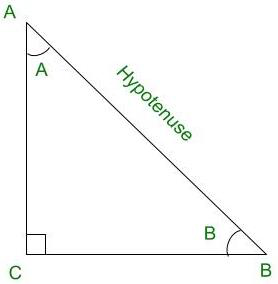# Find the value of sin75° + sin15°

• Last Updated : 03 Sep, 2021

Trigonometry is the relation between the angles and sides of a right-angled triangle. In a right-angled triangle, there are 3 angles of which one angle is a right angle (90°) and the other two angles are acute angles and there are 3 sides. The side opposite to the right angle is called Hypotenuse. There are 6 ratios between these sides based on the angle between them and they are called Trigonometric Ratios.

The 6 Trigonometric ratios are:

Hey! Looking for some great resources suitable for young ones? You've come to the right place. Check out our self-paced courses designed for students of grades I-XII

Start with topics like Python, HTML, ML, and learn to make some games and apps all with the help of our expertly designed content! So students worry no more, because GeeksforGeeks School is now here!

• Sine (sin)°
• Cosine (cos)
• Tangent (tan)
• Cosecant (cosec)
• Secant (sec)
• Cotangent (cot)Right-angled triangle ACB

Sine (sin):

Sine of an angle is defined by the ratio of lengths of sides which is opposite to the angle and the hypotenuse. For the above triangle, sin A = BC/AB

Cosine (cos):

Cosine of an angle is defined by the ratio of lengths of sides which is adjacent to the angle and the hypotenuse. For the above triangle, cos A = AC/AB

Tangent (tan):

Tangent of an angle is defined by the ratio of the length of sides which is opposite to the angle and the side which is adjacent to the angle. For the above triangle, tan A = BC/AC

Cosecant (cosec):

Cosecent of an angle is defined by the ratio of the length of the hypotenuse and the side opposite the angle. For the above triangle, cosec A = AB/BC

Secant (sec):

Secant of an angle is defined by the ratio of the length of the hypotenuse and the side and the side adjacent to the angle For the above triangle, sec A = AB/AC

Cotangent  (cot):

Cotangent  of an angle is defined by the ratio of length of sides which is adjacent to the angle and the side which is opposite to the angle. For the above triangle, cot A = AC/BC

### Find the value of sin75° + sin15°

Solution:

sin(75) + sin(15) = sin(45 + 30) + sin(45 – 30)

We know that, sin(A + B) = sinA.cosB + cosA.sinB and,

sin(A – B) = sinA.cosB – cosA.sinB

⇒ sin(75) + sin(15) = (sin45.cos30 + cos45.sin30) + (sin45.cos30 – cos45.sin30)

= 2sin45.cos30

= 2.(1/√2). (√3 / 2)

= √3/√2

⇒ sin(75) + sin(15) = √6/2

Therefore, the value of sin(75) + sin(15) is (√6 / 2).

### Similar Questions

Question 1: What is the value of sin(75) – sin(15).

Solution:

sin(75) – sin(15) = sin(45 + 30) – sin(45 – 30)

We know that, sin(A + B) = sinA.cosB + cosA.sinB and,

sin(A – B) = sinA.cosB – cosA.sinB

⇒ sin(75) – sin(15) = (sin45.cos30 + cos45.sin30) – (sin45.cos30 – cos45.sin30)

= 2cos45.sin30

= 2.(1/√2).(1 / 2)

= 1/√2

⇒ sin(75) – sin(15) = 1/√2

Therefore, the value of sin(75) – sin(15) is (1 / √2)

Question 2: What is the value of cos(75) + cos(15).

Solution:

cos(75) + cos(15) = cos(45 + 30) + cos(45 – 30)

We know that, cos(A + B) = cosA.cosB – sinA.sinB and,

cos(A – B) = cosA.cosB + sinA.sinB

⇒ cos(75) + cos(15) = (cos45.cos30 – sin45.sin30) + (cos45.cos30 + sin45.sin30)

= 2cos45.cos30

= 2.(1/√2).(√3 / 2)

= √3 / √2

⇒ cos(75) + cos(15) = √6/2

Therefore, the value of cos(75) + cos(15) is (√6 / 2).

Question 3: What is the value of cos(75) – cos(15).

Solution:

cos(75) – cos(15) = cos(45 + 30) – cos(45 – 30)

We know that, cos(A + B) = cosA.cosB – sinA.sinB and,

cos(A – B) = cosA.cosB + sinA.sinB

⇒ cos(75) – cos(15) = (cos45.cos30 – sin45.sin30) – (cos45.cos30 + sin45.sin30)

= – 2sin45.sin30

= – 2.(1/√2). (1 / 2)

= – 1/√2

⇒ cos(75) – cos(15) = – 1/√2

Therefore, the value of cos(75) – cos(15) is (– 1/ √2)

My Personal Notes arrow_drop_up